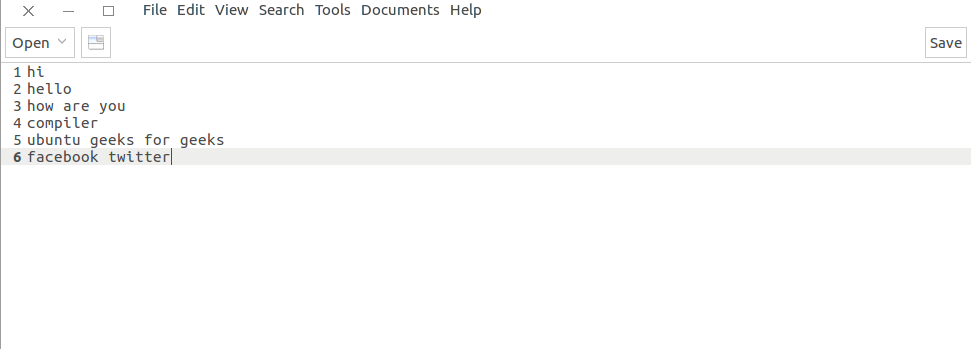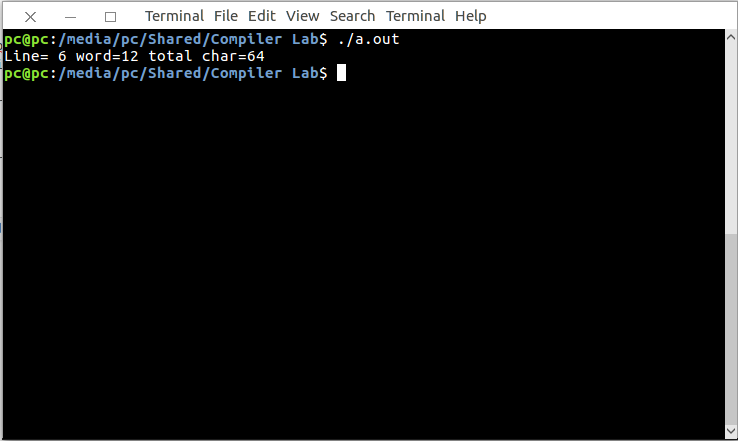# Lex Program to print the total characters, white spaces, tabs in the given input file

• Difficulty Level : Hard
• Last Updated : 30 Apr, 2019

Lex is a computer program that generates lexical analyzers. Lex reads an input stream specifying the lexical analyzer and outputs source code implementing the lexer in the C programming language.

The commands for executing the lex program are:

```lex abc.l (abc is the file name)
cc lex.yy.c -lfl
./a.out
```

Let’s see Lex program to print the total characters, white spaces, tabs in the given input file.

Below is the implementation:

 `/* Lex program to print the total characters, ``white spaces, tabs in the given input file.``*/`` ` `%``{``    ``int` `n, w, c;``    ``%``}``% %``\n { n++; }``[^ \n\t] +``{``    ``w++;``    ``c = c + yyleng;``}``.c++;``% % ``int` `yywrap(``void``)``{``    ``return` `1;``}`` ` ` ` `main()``{``    ``extern` `FILE``* yyin;``    ``yyin = ``fopen``(``"input.txt"``, ``"r"``);``    ``yylex();``    ``printf``(``"Line= %d word=%d total char=%d \n"``, n, w, c);``}`

Input:Output:My Personal Notes arrow_drop_up